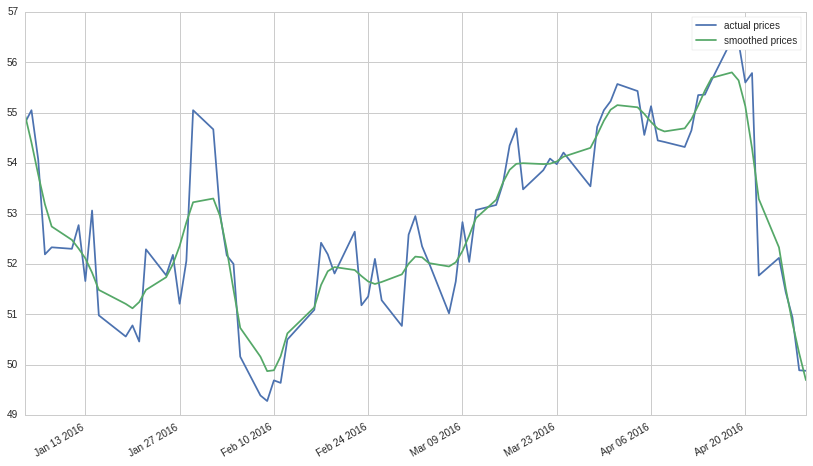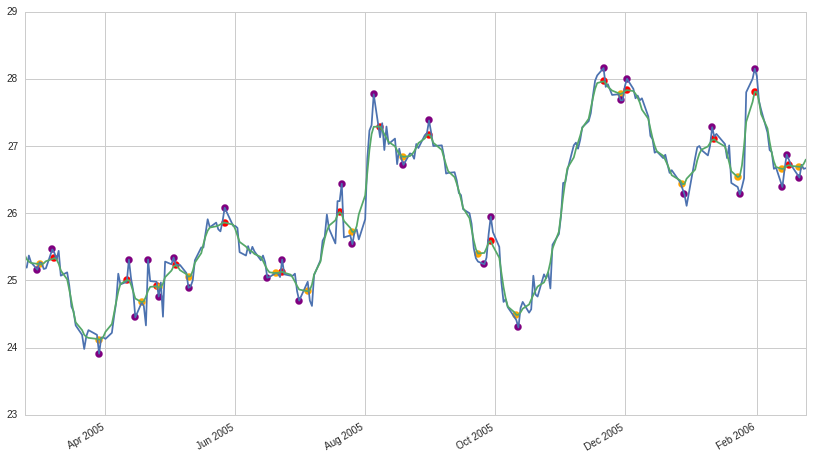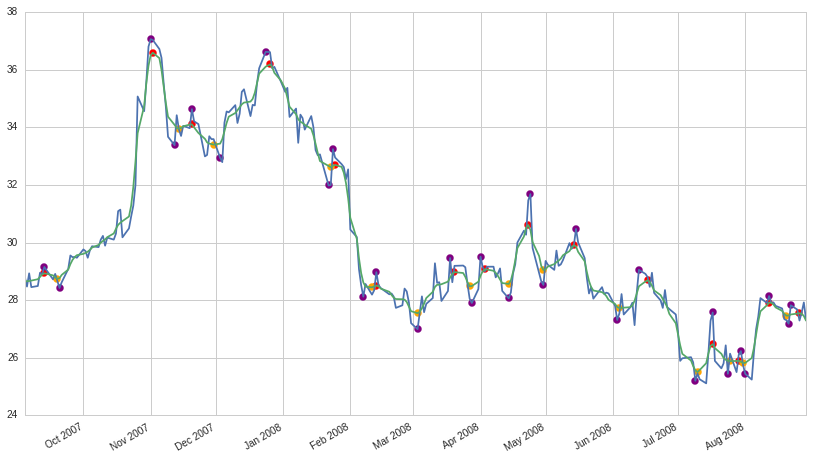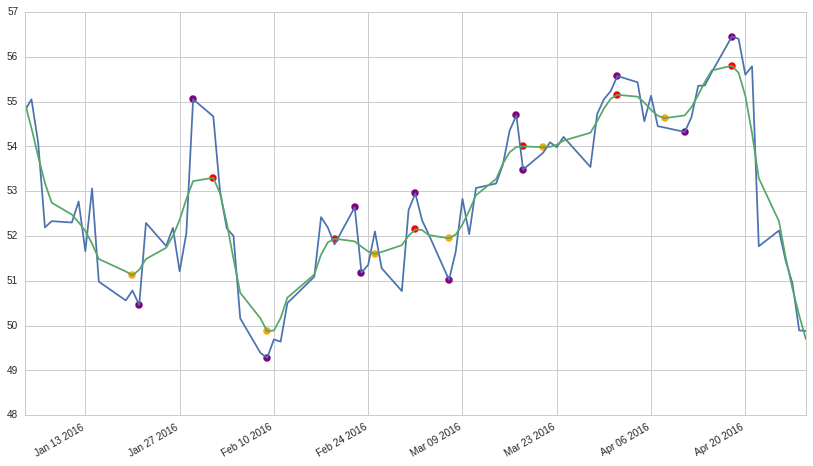Notebook

# Empirical Algorithmic Implementation of Technical Analysis¶

I recently presented a paper by Andrew Lo, Harry Mamaysky, and Jiang Wang called "Foundations of Technical Analysis: Computational Algorithms, Statistical Inference, and Empirical Implementation (2000)" at Quantopian's journal club. In the paper, the authors utilize non-parametric kernel regression to smooth a stock's daily price time series to a point where the local minima and maxima that a human technical analyst would find relevant can be separated from noisier short-term price fluctuations. The authors then search these denoised local minima and maxima for a few of the patterns commonly pursued by technical analysts. Once they've identified occurrences of particular patterns, the authors test their predictive power by observing the subsequent forward return on the stock.

For a fuller explanation of what is going on in this notebook, I encourage you to take a look at the original paper: https://www.cis.upenn.edu/~mkearns/teaching/cis700/lo.pdf

It is interesting to note that since this paper was written in 2000 and all the data used in my implementation is from 2003-2016, my results can be considered to be "out of sample" with respect to the authors' findings.

In :
from __future__ import division
import matplotlib.pyplot as plt
import pandas as pd
import numpy as np
from statsmodels.nonparametric.kernel_regression import KernelReg
from numpy import linspace
import seaborn as sns
from datetime import timedelta

In :
msft_prices = get_pricing('MSFT', start_date='2003-1-1', end_date='2016-5-1', fields='close_price')


In our application, a kernel regression estimates some function $m(x)$ given (time, price) observations $(x_1, P_1) ... (x_t, P_t)$ where $t = 1...T$. The value of $m(x)$ is computed by taking weighted average of the prices in the timeseries, where the weight assigned to observation $P_t$ is determinded by its distance from x. This distance weight is described by a "kernel" fuction $w(x)$. The authors use a gausian kernel with a manually tuned bandwidth parameter.

$$m(x) = \frac{1}{T}\sum_{t=1}^{T}w_t(x)P_t$$

In :
prices_ = msft_prices.copy()
prices_.index = linspace(1., len(prices_), len(prices_))

# I've chosen bandwith parameters manually. This is something that could be improved.
kr = KernelReg([prices_.values], [prices_.index.values], var_type='c', bw=[1.8,1])
f = kr.fit([prices_.index.values])
smooth_prices = pd.Series(data=f, index=msft_prices.index)

In :
msft_prices.loc[pd.datetime(2016,1,1):].plot()
smooth_prices.loc[pd.datetime(2016,1,1):].plot()
plt.legend(['actual prices', 'smoothed prices'])

Out:
<matplotlib.legend.Legend at 0x7f54fbbb17d0>From this smoothed price timeseries we can extract local minima and maxima (orange points in the plots below.)

The author use the minima and maxima from the smoothed timeseries to identify true local minima and maxima in the original timeseres by taking the maximum/minimum price within a t-1, t+1 window around the smooth timeseries maxima/minima (purple points in the plots below). We use these minima and maxima from the original price data to look for the given technical patterns.

You can see in the plots below, that finding minima and maxima using the kernel regression allows us to skip over minima and maxima that are too local.

In :
from scipy.signal import argrelextrema

In :
local_max = argrelextrema(smooth_prices.values, np.greater)
local_min = argrelextrema(smooth_prices.values, np.less)

In :
local_max_dt = smooth_prices.iloc[local_max].index.values
local_min_dt = smooth_prices.iloc[local_min].index.values

In :
price_local_max_dt = []
for i in local_max:
if (i>1) and (i<len(msft_prices)-1):
price_local_max_dt.append(msft_prices.iloc[i-2:i+2].argmax())

price_local_min_dt = []
for i in local_min:
if (i>1) and (i<len(msft_prices)-1):
price_local_min_dt.append(msft_prices.iloc[i-2:i+2].argmin())

In :
start = pd.datetime(2015,1,1)
end = pd.datetime(2015,4,1)

def plot_window(prices, smooth_prices, smooth_maxima_dt, smooth_minima_dt,
price_maxima_dt, price_minima_dt, start, end, ax=None):
if ax is None:
fig = plt.figure()

prices_ = prices.loc[start:end]
prices_.plot(ax=ax)
smooth_prices_ = smooth_prices.loc[start:end]
smooth_prices_.plot(ax=ax)

smooth_max = smooth_prices_.loc[smooth_maxima_dt]
smooth_min = smooth_prices_.loc[smooth_minima_dt]
price_max = prices_.loc[price_maxima_dt]
price_min = prices_.loc[price_minima_dt]

ax.scatter(smooth_max.index.values, smooth_max.values, s=50, color='red' )
ax.scatter(smooth_min.index.values, smooth_min.values, s=50, color='orange')

ax.scatter(price_max.index.values, price_max.values, s=50, color='purple')
ax.scatter(price_min.index.values, price_min.values, s=50, color='purple')

plot_window(msft_prices, smooth_prices, local_max_dt, local_min_dt, price_local_max_dt,
price_local_min_dt, pd.datetime(2005,2,19), pd.datetime(2006,2,26))
plot_window(msft_prices, smooth_prices, local_max_dt, local_min_dt, price_local_max_dt,
price_local_min_dt,pd.datetime(2007,9,1), pd.datetime(2008,9,1))
plot_window(msft_prices, smooth_prices, local_max_dt, local_min_dt, price_local_max_dt,
price_local_min_dt, pd.datetime(2016,1,1), pd.datetime(2016,5,1))In :
# Let's throw what we have so far into a function:
def find_max_min(prices):
prices_ = prices.copy()
prices_.index = linspace(1., len(prices_), len(prices_))
kr = KernelReg([prices_.values], [prices_.index.values], var_type='c', bw=[1.8,1])
f = kr.fit([prices_.index.values])
smooth_prices = pd.Series(data=f, index=prices.index)

local_max = argrelextrema(smooth_prices.values, np.greater)
local_min = argrelextrema(smooth_prices.values, np.less)

price_local_max_dt = []
for i in local_max:
if (i>1) and (i<len(prices)-1):
price_local_max_dt.append(prices.iloc[i-2:i+2].argmax())

price_local_min_dt = []
for i in local_min:
if (i>1) and (i<len(prices)-1):
price_local_min_dt.append(prices.iloc[i-2:i+2].argmin())

prices.name = 'price'
maxima = pd.DataFrame(prices.loc[price_local_max_dt])
minima = pd.DataFrame(prices.loc[price_local_min_dt])
max_min = pd.concat([maxima, minima]).sort_index()
max_min.index.name = 'date'
max_min = max_min.reset_index()
max_min = max_min[~max_min.date.duplicated()]
p = prices.reset_index()
max_min['day_num'] = p[p['index'].isin(max_min.date)].index.values
max_min = max_min.set_index('day_num').price

return max_min

In :
max_min = find_max_min(msft_prices)


# Pattern Identification¶

You can find the pattern definitions on page 1716 of the paper.

In :
from collections import defaultdict

def find_patterns(max_min):
patterns = defaultdict(list)

for i in range(5, len(max_min)):
window = max_min.iloc[i-5:i]

# pattern must play out in less than 36 days
if window.index[-1] - window.index > 35:
continue

# Using the notation from the paper to avoid mistakes
e1 = window.iloc
e2 = window.iloc
e3 = window.iloc
e4 = window.iloc
e5 = window.iloc

rtop_g1 = np.mean([e1,e3,e5])
rtop_g2 = np.mean([e2,e4])
if (e1 > e2) and (e3 > e1) and (e3 > e5) and \
(abs(e1 - e5) <= 0.03*np.mean([e1,e5])) and \
(abs(e2 - e4) <= 0.03*np.mean([e1,e5])):
patterns['HS'].append((window.index, window.index[-1]))

elif (e1 < e2) and (e3 < e1) and (e3 < e5) and \
(abs(e1 - e5) <= 0.03*np.mean([e1,e5])) and \
(abs(e2 - e4) <= 0.03*np.mean([e1,e5])):
patterns['IHS'].append((window.index, window.index[-1]))

elif (e1 > e2) and (e1 < e3) and (e3 < e5) and (e2 > e4):
patterns['BTOP'].append((window.index, window.index[-1]))

elif (e1 < e2) and (e1 > e3) and (e3 > e5) and (e2 < e4):
patterns['BBOT'].append((window.index, window.index[-1]))

# Triangle Top
elif (e1 > e2) and (e1 > e3) and (e3 > e5) and (e2 < e4):
patterns['TTOP'].append((window.index, window.index[-1]))

# Triangle Bottom
elif (e1 < e2) and (e1 < e3) and (e3 < e5) and (e2 > e4):
patterns['TBOT'].append((window.index, window.index[-1]))

# Rectangle Top
elif (e1 > e2) and (abs(e1-rtop_g1)/rtop_g1 < 0.0075) and \
(abs(e3-rtop_g1)/rtop_g1 < 0.0075) and (abs(e5-rtop_g1)/rtop_g1 < 0.0075) and \
(abs(e2-rtop_g2)/rtop_g2 < 0.0075) and (abs(e4-rtop_g2)/rtop_g2 < 0.0075) and \
(min(e1, e3, e5) > max(e2, e4)):

patterns['RTOP'].append((window.index, window.index[-1]))

# Rectangle Bottom
elif (e1 < e2) and (abs(e1-rtop_g1)/rtop_g1 < 0.0075) and \
(abs(e3-rtop_g1)/rtop_g1 < 0.0075) and (abs(e5-rtop_g1)/rtop_g1 < 0.0075) and \
(abs(e2-rtop_g2)/rtop_g2 < 0.0075) and (abs(e4-rtop_g2)/rtop_g2 < 0.0075) and \
(max(e1, e3, e5) > min(e2, e4)):
patterns['RBOT'].append((window.index, window.index[-1]))

return patterns

In :
patterns = find_patterns(max_min)


Visualizing pattern occurences

In :
for name, end_day_nums in patterns.iteritems():
print(name)
rows = int(np.ceil(len(end_day_nums)/2))
f, axes = plt.subplots(rows,2, figsize=(20,5*rows))
axes = axes.flatten()
i = 0
for sd, ed in end_day_nums:
s = msft_prices.index[sd-1]
e = msft_prices.index[ed+1]
plot_window(msft_prices, smooth_prices,local_max_dt, local_min_dt,
price_local_max_dt, price_local_min_dt,
s, e, ax=axes[i])
i+=1
plt.show()

HS